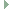OGRE-Next  2.3 Object-Oriented Graphics Rendering Engine
Ogre::Plane Class Reference

Defines a plane in 3D space. More...

`#include <OgrePlane.h>`Inheritance diagram for Ogre::Plane:

## Public Types

enum  Side { NO_SIDE , POSITIVE_SIDE , NEGATIVE_SIDE , BOTH_SIDE }
The "positive side" of the plane is the half space to which the plane normal points. More...

## Public Member Functions

Plane ()
Default constructor - sets everything to 0. More...

Plane (const Vector3 &p0, const Vector3 &p1, const Vector3 &p2)

Plane (const Vector3 &rkNormal, const Vector3 &rkPoint)

Plane (const Vector3 &rkNormal, Real fConstant)
Construct a plane through a normal, and a distance to move the plane along the normal. More...

Plane (Real a, Real b, Real c, Real _d)
Construct a plane using the 4 constants directly. More...

Real getDistance (const Vector3 &rkPoint) const
This is a pseudodistance. More...

Side getSide (const AxisAlignedBox &rkBox) const
Returns the side where the alignedBox is. More...

Side getSide (const Vector3 &centre, const Vector3 &halfSize) const
Returns which side of the plane that the given box lies on. More...

Side getSide (const Vector3 &rkPoint) const

Real normalise ()
Normalises the plane. More...

bool operator!= (const Plane &rhs) const

Plane operator- () const
Get flipped plane, with same location but reverted orientation. More...

bool operator== (const Plane &rhs) const
Comparison operator. More...

Vector3 projectVector (const Vector3 &v) const
Project a vector onto the plane. More...

void redefine (const Vector3 &p0, const Vector3 &p1, const Vector3 &p2)
Redefine this plane based on 3 points. More...

void redefine (const Vector3 &rkNormal, const Vector3 &rkPoint)
Redefine this plane based on a normal and a point. More...

Real d

Vector3 normal

## Friends

_OgreExport friend std::ostream & operator<< (std::ostream &o, const Plane &p)

## Detailed Description

Defines a plane in 3D space.

Remarks
A plane is defined in 3D space by the equation Ax + By + Cz + D = 0
This equates to a vector (the normal of the plane, whose x, y and z components equate to the coefficients A, B and C respectively), and a constant (D) which is the distance along the normal you have to go to move the plane back to the origin.

## ◆ Side

 enum Ogre::Plane::Side

The "positive side" of the plane is the half space to which the plane normal points.

The "negative side" is the other half space. The flag "no side" indicates the plane itself.

Enumerator
NO_SIDE
POSITIVE_SIDE
NEGATIVE_SIDE
BOTH_SIDE

## ◆ Plane() [1/5]

 Ogre::Plane::Plane ( )
inline

Default constructor - sets everything to 0.

## ◆ Plane() [2/5]

 Ogre::Plane::Plane ( const Vector3 & rkNormal, Real fConstant )
inline

Construct a plane through a normal, and a distance to move the plane along the normal.

## ◆ Plane() [3/5]

 Ogre::Plane::Plane ( Real a, Real b, Real c, Real _d )
inline

Construct a plane using the 4 constants directly.

## ◆ Plane() [4/5]

 Ogre::Plane::Plane ( const Vector3 & rkNormal, const Vector3 & rkPoint )
inline

## ◆ Plane() [5/5]

 Ogre::Plane::Plane ( const Vector3 & p0, const Vector3 & p1, const Vector3 & p2 )
inline

## ◆ getDistance()

 Real Ogre::Plane::getDistance ( const Vector3 & rkPoint ) const
inline

This is a pseudodistance.

The sign of the return value is positive if the point is on the positive side of the plane, negative if the point is on the negative side, and zero if the point is on the plane.

The absolute value of the return value is the true distance only when the plane normal is a unit length vector.

References Ogre::Vector3::dotProduct().

Referenced by Ogre::PlaneBoundedVolume::intersects().

## ◆ getSide() [1/3]

 Side Ogre::Plane::getSide ( const AxisAlignedBox & rkBox ) const

Returns the side where the alignedBox is.

The flag BOTH_SIDE indicates an intersecting box. One corner ON the plane is sufficient to consider the box and the plane intersecting.

## ◆ getSide() [2/3]

 Side Ogre::Plane::getSide ( const Vector3 & centre, const Vector3 & halfSize ) const

Returns which side of the plane that the given box lies on.

The box is defined as centre/half-size pairs for effectively.

Parameters
 centre The centre of the box. halfSize The half-size of the box.
Returns
POSITIVE_SIDE if the box complete lies on the "positive side" of the plane, NEGATIVE_SIDE if the box complete lies on the "negative side" of the plane, and BOTH_SIDE if the box intersects the plane.

## ◆ getSide() [3/3]

 Side Ogre::Plane::getSide ( const Vector3 & rkPoint ) const
inline

## ◆ normalise()

 Real Ogre::Plane::normalise ( )

Normalises the plane.

Remarks
This method normalises the plane's normal and the length scale of d is as well.
Note
This function will not crash for zero-sized vectors, but there will be no changes made to their components.
Returns
The previous length of the plane's normal.

## ◆ operator!=()

 bool Ogre::Plane::operator!= ( const Plane & rhs ) const
inline

References d, and normal.

## ◆ operator-()

 Plane Ogre::Plane::operator- ( ) const
inline

Get flipped plane, with same location but reverted orientation.

References Ogre::Vector3::x, Ogre::Vector3::y, and Ogre::Vector3::z.

## ◆ operator==()

 bool Ogre::Plane::operator== ( const Plane & rhs ) const
inline

Comparison operator.

References d, and normal.

## ◆ projectVector()

 Vector3 Ogre::Plane::projectVector ( const Vector3 & v ) const

Project a vector onto the plane.

Remarks
This gives you the element of the input vector that is perpendicular to the normal of the plane. You can get the element which is parallel to the normal of the plane by subtracting the result of this method from the original vector, since parallel + perpendicular = original.
Parameters
 v The input vector

## ◆ redefine() [1/2]

 void Ogre::Plane::redefine ( const Vector3 & p0, const Vector3 & p1, const Vector3 & p2 )
inline

Redefine this plane based on 3 points.

References Ogre::Vector3::dotProduct(), and Ogre::Vector3::normalisedCopy().

## ◆ redefine() [2/2]

 void Ogre::Plane::redefine ( const Vector3 & rkNormal, const Vector3 & rkPoint )
inline

Redefine this plane based on a normal and a point.

References Ogre::Vector3::dotProduct().

## ◆ operator<<

 _OgreExport friend std::ostream& operator<< ( std::ostream & o, const Plane & p )
friend

## ◆ d

 Real Ogre::Plane::d

## ◆ normal

 Vector3 Ogre::Plane::normal

The documentation for this class was generated from the following file: